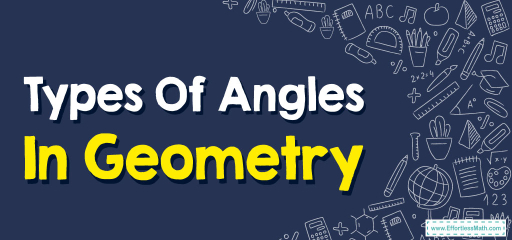# Types Of Angles In Geometry

Angles are everywhere – from the corners of a book to the bend of an elbow. They define the space between two lines or rays and play a crucial role in both basic and advanced geometry. While the concept might seem elementary, understanding the different types of angles is essential as it serves as a foundation for various geometric principles and real-world applications. Dive into this guide to explore the diverse world of angles and their distinct characteristics.## Step-by-step Guide: Types of Angles

Acute Angle:
An angle that measures less than $$90^\circ$$ is termed as an acute angle. It’s like a slightly opened book or the sharp tip of a slice of pie.
Representation: If $$\angle ABC$$ measures $$45^\circ$$, then $$\angle ABC$$ is an acute angle.

Right Angle:
A right angle measures exactly $$90^\circ$$. Think of the corners of a rectangular book or the intersection of two perpendicular lines.
Representation: The angle is often marked by a small square at the intersection, signifying its $$90^\circ$$ nature. If $$\angle DEF$$ is a right angle, it measures $$90^\circ$$.

Obtuse Angle:
An obtuse angle measures more than $$90^\circ$$ but less than $$180^\circ$$. It’s wider than a right angle but doesn’t stretch out flat. Picture a largely opened book.
Representation: If $$\angle GHI$$ measures $$120^\circ$$, then $$\angle GHI$$ is an obtuse angle.

Straight Angle:
A straight angle measures exactly $$180^\circ$$. Imagine a straight line or the flat edge of a ruler.
Representation: If rays $$BA$$ and $$BC$$ lie on the same straight line with point $$B$$ as their endpoint, then $$\angle ABC$$ is a straight angle.

Reflex Angle:
A reflex angle measures more than $$180^\circ$$ but less than $$360^\circ$$. It’s the larger angle formed when you have an obtuse or acute angle, representing the space ‘outside’ the smaller angle.
Representation: If $$\angle JKL$$ measures $$270^\circ$$, then $$\angle JKL$$ is a reflex angle.

Complete Angle:
A complete angle measures $$360^\circ$$. Think of a full circle or a clock’s hands making a complete round.
Representation: If rays $$MA$$ and $$MC$$ start and end in the same position after a full rotation around $$M$$, then $$\angle AMC$$ is a complete angle.

### Examples

Example 1:
Three angles – one at the tip of a triangle, another at the corner of a square, and the last one representing almost three-fourths of a circle.

Solution:
The angle at the tip of the triangle is acute, the one at the square’s corner is a right angle, and the near-complete circular one is a reflex angle.

Example 2:
You’re setting up a sun dial. The angle between the gnomon (the dial’s part that casts the shadow) and the base needs to be what type?

Solution:
The gnomon should make a right angle with the base for accurate time-telling.

### Practice Questions:

1. If the hands of a clock are at $$3$$ and $$9$$, what type of angle do they form?
2. What type of angle is formed when the hands of a clock read 2:30?
3. When you partially open a door, what type of angle is most commonly formed between the door and its frame?

1. The hands form a straight angle of $$180^\circ$$.
2. They form an acute angle as the minute hand on 6 and the hour hand is halfway between $$2$$ and $$3$$, resulting in an angle less than $$90^\circ$$.
3. Most commonly, it’s an obtuse angle as doors, when partially open, usually create an angle larger than $$90^\circ$$ but less than $$180^\circ$$.

### What people say about "Types Of Angles In Geometry - Effortless Math: We Help Students Learn to LOVE Mathematics"?

No one replied yet.

X
30% OFF

Limited time only!

Save Over 30%

SAVE $5 It was$16.99 now it is \$11.99